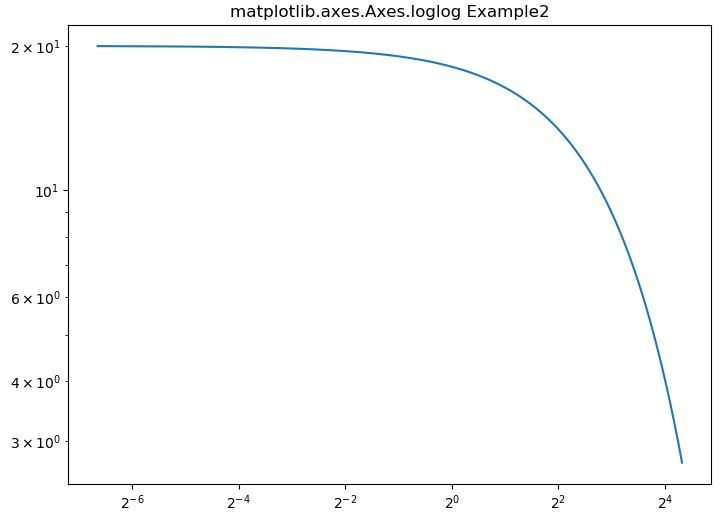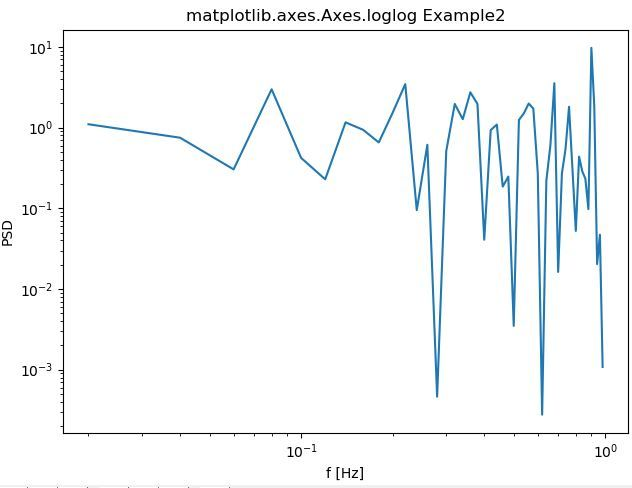# matplotlib.axes.Axes.loglog() in Python

• Last Updated : 13 Apr, 2020

Matplotlib is a library in Python and it is numerical – mathematical extension for NumPy library. The Axes Class contains most of the figure elements: Axis, Tick, Line2D, Text, Polygon, etc., and sets the coordinate system. And the instances of Axes supports callbacks through a callbacks attribute.

## matplotlib.axes.Axes.loglog() Function

The Axes.errorbar() function in axes module of matplotlib library is used to make a plot with log scaling on both the x and y axis.

Syntax:

`Axes.loglog(self, *args, **kwargs)`

Parameters: This method accept the following parameters that are described below:

• basex, basey: These parameter are Base of the x/y logarithm and are optional with default value 10.
• subsx, subsy: These parameter are the sequence of location of the minor x/y ticks and are optional.
• nonposx, nonposy: These parameter are non-positive values in x or y that can be masked as invalid, or clipped to a very small positive number.

Returns: This returns the following:

• lines:This returns the list of Line2D objects representing the plotted data..

Below examples illustrate the matplotlib.axes.Axes.loglog() function in matplotlib.axes:

Example-1:

 `# Implementation of matplotlib function``     ` `import` `numpy as np``import` `matplotlib.pyplot as plt`` ` `t ``=` `np.arange(``0.01``, ``20.0``, ``0.01``)`` ` `# Create figure``fig, ax ``=` `plt.subplots()`` ` `# log x and y axis``ax.loglog(t, ``20` `*` `np.exp(``-``t ``/` `10.0``), basex ``=` `2``)`` ` `ax.set_title(``'matplotlib.axes.Axes.loglog Example2'``)``plt.show()`

Output:Example-2:

 `# Implementation of matplotlib function``     ` `import` `numpy as np``import` `matplotlib.pyplot as plt`` ` `fig, ax ``=` `plt.subplots(constrained_layout ``=` `True``)``x ``=` `np.arange(``0.02``, ``1``, ``0.02``)``np.random.seed(``19680801``)``y ``=` `np.random.randn(``len``(x)) ``*``*` `2``ax.loglog(x, y)``ax.set_xlabel(``'f [Hz]'``)``ax.set_ylabel(``'PSD'``)``ax.set_title(``'Random spectrum'``)`` ` ` ` `def` `forward(x):``    ``return` `1` `/` `x`` ` ` ` `def` `inverse(x):``    ``return` `1` `/` `x`` ` `ax.set_title(``'matplotlib.axes.Axes.loglog Example2'``)``plt.show()`

Output:My Personal Notes arrow_drop_up